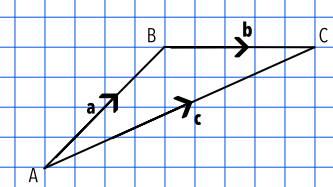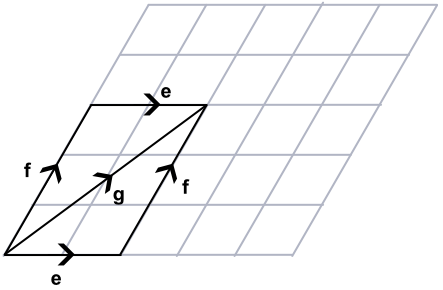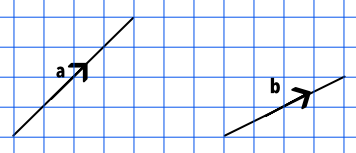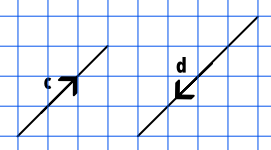Vectors can be added and subtracted.Let vec(AB) = bb(a) = ((4),(4)), vec(BC) = bb(b) = ((5),(0)), and vec(AC) = bb(c) = ((9),(4))

Start at A and move along vector a to point B. Then move to point C using vector b. This is equivalent to moving directly from A to C using vector c.

bb(c) = bb(a) + bb(b) = ((4 + 5),(4 + 0)) = ((9),(4))

This is the Triangle Law for Vector Addition. This shows that vectors can be added, and from that they can also be subtracted, multiplied and divided.

The Parallelogram Law for Vector Addition states that the diagonal vector, g, is equal to f + e. The diagonal vector is called the resultant vector. You can get the same resultant vector by adding the vectors in the other order. This shows that adding vectors is commutative: it does not matter which way round the vectors are added:

bb(a) + bb(b) = bb(b) + bb(a)## Example 1

Write a + b as a column vector:a = ((4),(4)) and b = ((4),(2))

((4),(4)) + ((4),(2)) = ((8),(6))

Answer: ((8),(6))

## Example 2

g = c - d. Write g as a column vector.g = ((3),(3)) - ((-4),(-4)) = ((7),(7))

Answer: ((7),(7))